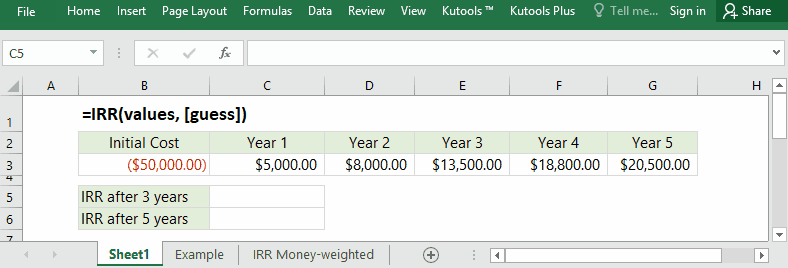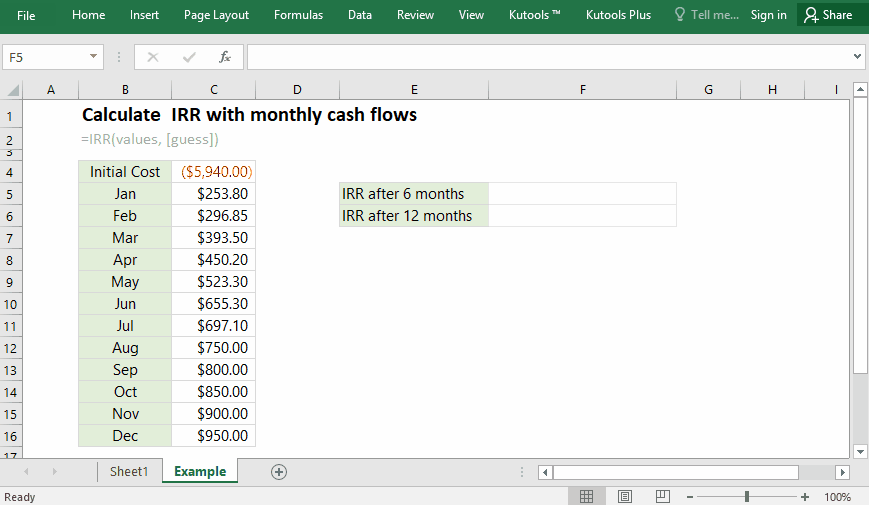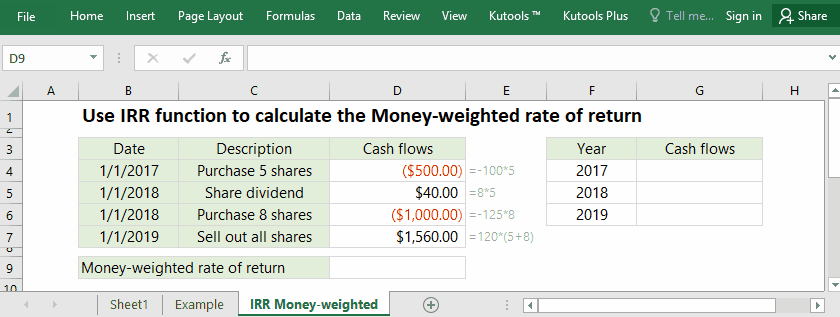## Excel IRR Function

The IRR, internal rate of return, is an important factor to judge the feasibility of a project. In usual, we can set the NPV (net present value) to 0, and figure out the discount rate with the trial-and-error approach. Now, you can apply the IRR function to calculate the IRR easily in Excel.#### Function syntax and arguments

IRR(values, [guess])

(1) Values: Required. They are the cash flow values that you will calculate the IRR for.

It can be an array such as {-50000, 5000, 8000, 13500, 18800, 20500}, or a reference of cells such as B3:G3.
Values must consist of cash out (negative values) and cash received (positive values).

(2) Guess: Optional. It’s an estimate number close to the result of IRR. If omitted, it will be assumed to 0.1 (10%).

#### Return Value

Numeric value.

The IRR function returns the internal rate of return for a series of cash flows that occur at regular intervals, says monthly or annually.

#### Usage notes

(1) The IRR function will ignore text values, logical values, or empty cells in the values argument.

(2) If the IRR function returns the #NUM! error value, or the calculation result is far from the expected interest rate, you can add or change the guess argument.

(3) The IRR function returns the interval rate of return for a period. You can use the formula =(1+r)^n -1 to convert the IRR to annual internal rate of return.

• For monthly stream of cash flows, the IRR function will return the monthly interval rate of return (says 0.5%), and you can convert it to annual rate 6.17% (formula: =(1+0.5%)^12-1);
• For quarterly stream of cash flows, the IRR function will return the quarterly interval rate of return (says 1.5%), and you can convert it to annual rate 6.14% (formula: =(1+1.5%)^4-1);
• If you list your cash flows every half year, the IRR function will return the semi-annual interval rate of return (says 3%), and you can convert it to annual rate 6.09% (formula: =(1+3%)^2-1)

#### Formula Examples

##### Example 1: Calculate IRR with monthly cash flows in Excel

Supposing you will spend \$5,940 to get a power mower at the beginning of this year, and then rent it out. With the forecast rental in each month, you can easily figure out the IRR (internal rate of return), and judge the feasibility of this project.For calculating the IRR after 6 months, the values are cash flows in Range C4:C10, therefore you can apply one of below formulas:

=IRR(C4:C10)

=IRR({-5940,253.8,296.85,393.5,450.2,523.3,655.3})

For calculating the IRR after 12 months, the values are cash flows in the Range C4:C16, therefore you can apply one of below formulas:

=IRR(C4:C16)

=IRR({-5940,253.8,296.85,393.5,450.2,523.3,655.3,697.1,750,800,850,900,950})

Tips:
1. In Cell C4, the initial cost should be entered as negative number: -5940.00 as it is the cash you pay out.
2. In this example, the cash flows are listed by month, therefore the IRR function returns the monthly internal rate of return. If you need to return an annual rate, you can use this formula:

=POWER((IRR(C4:C16)+1),12)-1

##### Example 2: Use IRR function to calculate the Money-weighted rate of return in Excel

Let’s say you purchased 5 shares of Company A with the price of \$100 per share on 2017/1/1. And then you bought 8 shares of the Company A with price of \$125 per share again on 2018/1/1. You received a per-share dividend of \$8 on 2018/1/1 too. Finally, you decided to sell all shares of the Company A with the price of \$120 per share on 2019/1/1. In this case, you can figure out the money-weighted rate of return with the IRR function easily in Excel.In this case, the cash flows of 3 years can be calculated as follows:
(1) In Year 2017, you paid \$500 out (=-100*5);
(2) In Year 2018, you received share dividend \$40 (=5*8), and paid \$1,000 (=-125*8) for purchasing 8 shares, so the total cash flow is -\$960 (=-1000+40);
(3) In Year 2019, you received \$1560 (=120*(5+8));

Therefore, you can apply one of below formulas to figure out the money-weighted rate of return.

=IRR({-500,-960,1560})

=IRR(G4:G6)

### The Best Office Productivity Tools

#### Kutools for Excel - Helps You To Stand Out From Crowd

 Popular Features: Find, Highlight or Identify Duplicates  |  Delete Blank Rows  |  Combine Columns or Cells without Losing Data  |  Round without Formula ... Super VLookup: Multiple Criteria  |  Multiple Value  |  Across Multi-Sheets  |  Fuzzy Lookup... Adv. Drop-down List: Easy Drop Down List  |  Dependent Drop Down List  |  Multi-select Drop Down List... Column Manager: Add a Specific Number of Columns  |  Move Columns  |  Toggle Visibility Status of Hidden Columns  |  Compare Columns to Select Same & Different Cells ... Featured Features: Grid Focus  |  Design View  |  Big Formula Bar  |  Workbook & Sheet Manager | Resource Library (Auto Text)  |  Date Picker  |  Combine Worksheets  |  Encrypt/Decrypt Cells  |  Send Emails by List  |  Super Filter  |  Special Filter (filter bold/italic/strikethrough...) ... Top 15 Toolsets:  12 Text Tools (Add Text, Remove Characters ...)  |  50+ Chart Types (Gantt Chart ...)  |  40+ Practical Formulas (Calculate age based on birthday ...)  |  19 Insertion Tools (Insert QR Code, Insert Picture from Path ...)  |  12 Conversion Tools (Numbers to Words, Currency Conversion ...)  |  7 Merge & Split Tools (Advanced Combine Rows, Split Excel Cells ...)  |  ... and more

Kutools for Excel Boasts Over 300 Features, Ensuring That What You Need is Just A Click Away...#### Office Tab - Enable Tabbed Reading and Editing in Microsoft Office (include Excel)

• One second to switch between dozens of open documents!
• Reduce hundreds of mouse clicks for you every day, say goodbye to mouse hand.
• Increases your productivity by 50% when viewing and editing multiple documents.
• Brings Efficient Tabs to Office (include Excel), Just Like Chrome, Edge and Firefox.